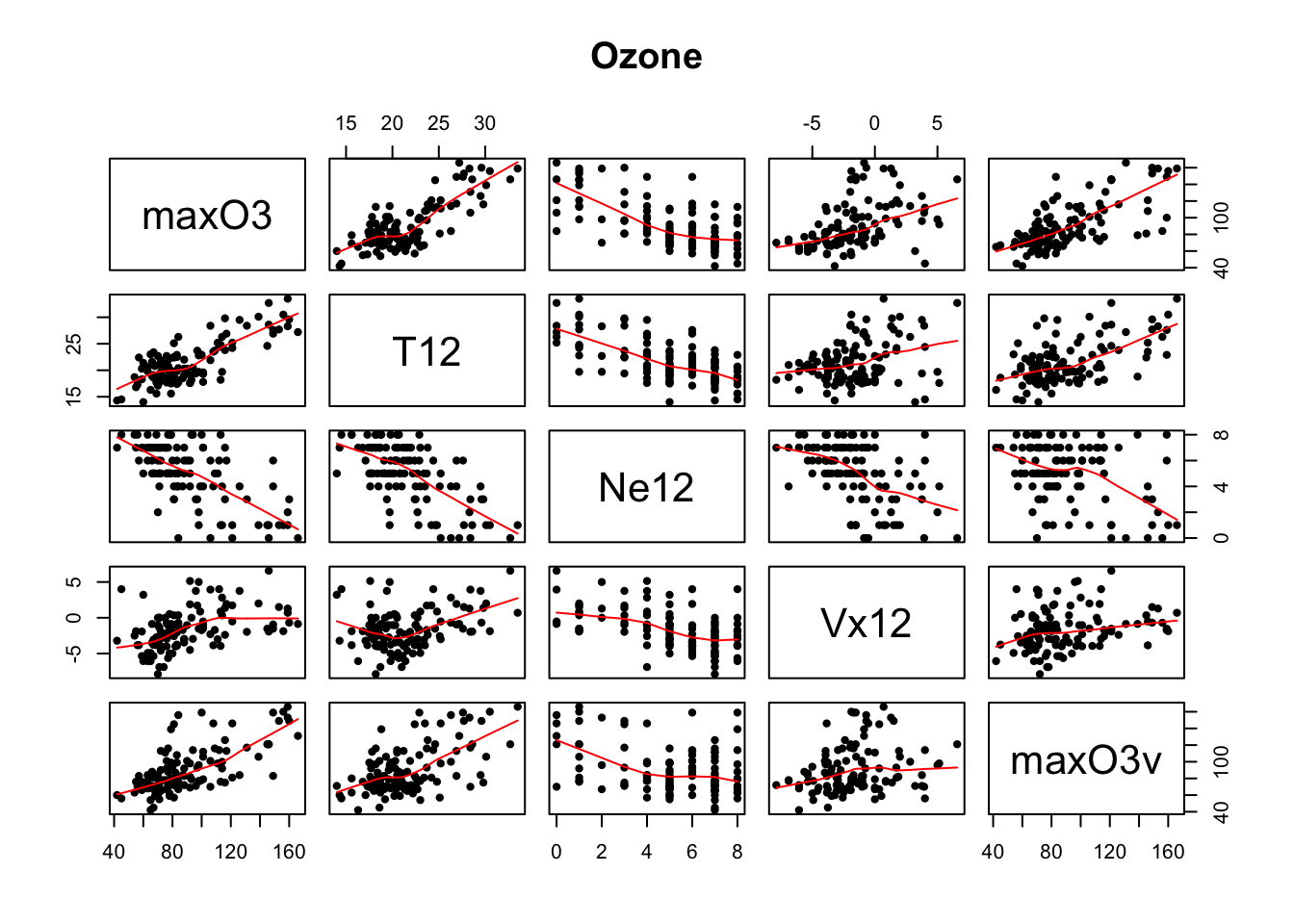## Ozone - max concentration

``````Ozone = read.table("https://perso.univ-rennes1.fr/valerie.monbet/MachineLearning/Ozone.dat",header=TRUE,row.names=1)
pairs(Ozone[,c(1,3,6,9,11)], panel = panel.smooth, main = "Ozone",pch=20)``````## Gaussian graphical models

First fit a graphical model on a subset of the variables. The regularizaiton constant is chosen to be equal to 0.5. You can try to change it.

``require(glasso)``
``## Loading required package: glasso``
``require(qgraph)``
``## Loading required package: qgraph``
``require(fields)``
``## Loading required package: fields``
``## Loading required package: spam``
``## Loading required package: dotCall64``
``## Loading required package: grid``
``````## Spam version 2.1-1 (2017-07-02) is loaded.
## Type 'help( Spam)' or 'demo( spam)' for a short introduction
## and overview of this package.
## Help for individual functions is also obtained by adding the
## suffix '.spam' to the function name, e.g. 'help( chol.spam)'.``````
``````##
## Attaching package: 'spam'``````
``````## The following objects are masked from 'package:base':
##
##     backsolve, forwardsolve``````
``## Loading required package: maps``
``````C.ozone = cor(Ozone[,c(1,3,6,9)])
a = glasso(C.ozone,.5) #
qgraph(a, layout="spring",
labels=names(Ozone[,c(1,3,6,9)]), label.scale=FALSE,
label.cex=1, node.width=1)``````